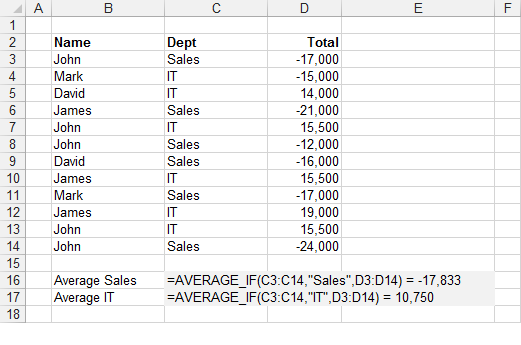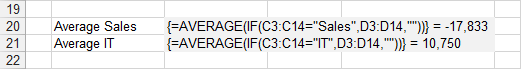### AVERAGEIF

Returns the average of a range of values that match a certain condition.

`'rgeCriteria - The range of cells containing the criteria you want to check.'sCriteria - The criteria value you want to match.'rgeAverageRange - The range of corresponding values you want to average.Public Function AVERAGE_IF(ByVal rgeCriteria As Range, _                            ByVal sCriteria As String, _                   Optional ByVal rgeAverageRange As Range = Nothing) As Single           Dim iconditionno As Integer Dim inumbersno As Integer Dim lrowno As Long Dim lcolno As Long Dim lmatch As Long Dim sngaverage As Single Dim vcellvalue As Variant               Call Application.Volatile(True)               If (rgeAverageRange Is Nothing) Then Set rgeAverageRange = rgeCriteria               'Data arranged in a column    If (rgeCriteria.Rows.Count > rgeCriteria.Columns.Count) Then         iconditionno = rgeCriteria.Column         inumbersno = rgeAverageRange.Column                  For lrowno = 1 To rgeCriteria.Rows.Count            vcellvalue = rgeCriteria.Parent.Cells(rgeCriteria.Row + lrowno - 1, inumbersno).Value            If (IsNumeric(vcellvalue) = True And IsEmpty(vcellvalue) = False) Then               If (Criteria_Check(sCriteria, rgeCriteria.Parent.Cells(rgeCriteria.Row + lrowno - 1, iconditionno).Value)) Then                  lmatch = lmatch + 1                  sngaverage = sngaverage + rgeCriteria.Parent.Cells(rgeCriteria.Row + lrowno - 1, inumbersno).Value               End If            End If         Next lrowno     End If         'Data arranged in a row    If (rgeCriteria.Columns.Count > rgeCriteria.Rows.Count) Then         iconditionno = rgeCriteria.Row         inumbersno = rgeAverageRange.Row         For lcolno = 1 To rgeCriteria.Columns.Count            vcellvalue = rgeCriteria.Parent.Cells(inumbersno, rgeCriteria.Column + lcolno - 1).Value            If (IsNumeric(vcellvalue) = True And IsEmpty(vcellvalue) = False) Then               If (Criteria_Check(sCriteria, rgeCriteria.Parent.Cells(iconditionno, rgeCriteria.Column + lcolno - 1).Value)) Then                  lmatch = lmatch + 1                  sngaverage = sngaverage + rgeCriteria.Parent.Cells(inumbersno, rgeCriteria.Column + lcolno - 1).Value               End If            End If         Next lcolno     End If         AVERAGE_IF = sngaverage / lmatch End Function Public Function Criteria_Check(ByVal sCriteria As String, _                                ByVal sValue As String) As Boolean         If ((InStr(sCriteria, "*") > 0) And (InStr(sCriteria, "~*") = 0)) Or _        ((InStr(sCriteria, "?") > 0) And (InStr(sCriteria, "~?") = 0)) Then         Criteria_Check = (sValue Like sCriteria)         Exit Function     End If     If (Left(sCriteria, 1) = "=") Then         Criteria_Check = (sValue = Right(sCriteria, Len(sCriteria) - 1))         Exit Function     End If     If (Left(sCriteria, 1) = ">") And _        (Left(sCriteria, 2) <> ">=") Then         Criteria_Check = (sValue > Right(sCriteria, Len(sCriteria) - 1))         Exit Function     End If     If (Left(sCriteria, 1) = "<") And _        (Left(sCriteria, 2) <> "<=") And (Left(sCriteria, 2) <> "<>") Then         Criteria_Check = (sValue < Right(sCriteria, Len(sCriteria) - 1))         Exit Function     End If     If (Left(sCriteria, 2) = ">=") Then         Criteria_Check = (sValue >= Right(sCriteria, Len(sCriteria) - 2))         Exit Function     End If     If (Left(sCriteria, 2) = "<=") Then         Criteria_Check = (sValue <= Right(sCriteria, Len(sCriteria) - 2))         Exit Function     End If     If (Left(sCriteria, 2) = "<>") Then         Criteria_Check = (sValue <> Right(sCriteria, Len(sCriteria) - 2))         Exit Function     End If     Criteria_Check = sValue Like sCriteria End Function `

AVERAGEIF was added as a built-in function in 2007.
AVERAGEIFS was added as a built-in function in 2007.
There is an Array Formula equivalent if you do not want to use the user defined function (see below).
This includes hidden rows.
This user defined function is similar to the built-in SUMIF function.#### Array Formula

To find out more about Array Formulas please refer to the Array Formulas section.
To find out more about the individual functions please refer to the AVERAGE function and IF function pages.
This example illustrates how to use an array formula to obtain the average value in a column based on a condition.The formula in row 16 returns the average in the "Sales" department.
The formula in row 17 returns the average in the "IT" department.
The formula [C3:C14="Sales"] returns an array of 1's and 0's indicating if the value in column "C" satisfies the condition.
Every number 1 in this array represents the value True in the IF statement and therefore has its corresponding value returned from column "D".
Every number 0 in this array represents the value False in the IF statement and therefore an empty string is returned in this case. The MAX function will ignore any string values.
The use of the empty string is to ensure that the formula works with both positive and negative numbers.
This array of numbers is then passed to the AVERAGE function to obtain the largest number.
Remember that you must press (Ctrl + Shift + Enter) to enter the formulas.
You can also use the SUMPRODUCT Function

#### Important

You cannot use column references (such as "A:A" or "D:D") in your array formulas.
Any cell ranges passed to an array formula must have the same number of rows. This is to ensure that all the temporary arrays used are the same length.